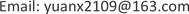﻿ 课堂教学评估体系与方法研究 Research on Evaluation System and Method of Classroom Teaching

Vol. 08  No. 07 ( 2019 ), Article ID: 31452 , 9 pages
10.12677/ASS.2019.87174

Research on Evaluation System and Method of Classroom Teaching

Wen Cheng, Xiang Yuan

School of Economics & Management, Shanghai Maritime University, ShanghaiReceived: Jul. 3rd, 2019; accepted: Jul. 17th, 2019; published: Jul. 26th, 2019ABSTRACT

In the new situation of university competition, how to improve the quality of teaching and enhance the competitiveness of the school has become an important subject of each university. So the establishment of classroom teaching evaluation system and evaluation methods will have a vital significance. In this paper, AHP is used to establish the hierarchical relationship of the classroom teaching quality evaluation index system, and the weight vector and the weight vector are obtained, and the weights under different evaluation systems are obtained. Finally, through the established model, some reasonable suggestions are given to the teacher’s classroom teaching, so as to improve teaching methods and improve teaching quality.

Keywords:Classroom Teaching Evaluation System, Evaluation Method, Analytic Hierarchy Process1. 引言

2. 课堂教学评估体系的确立与实例分析(层次分析法与模糊综合评价)

2.1. 层次分析法模型的实例分析

1) 学生评教指标体系

2) 同行教师评教指标体系Table 1. Students’ evaluation index system for classroom teaching qualityTable 2. Peer-to-teacher evaluation index system for classroom teaching quality

3) 专家组评教指标体系Table 3. Expert group’s evaluation index system for classroom teaching quality

2.1.1. 构造影响课堂教学质量的判断矩阵

${a}_{ij}=\frac{1}{{a}_{ji}}$ (1)

${a}_{ii}=1$ (2)

2.1.2. 计算判断矩阵的相对权重系数并进行一致性检验

1) 相对权重系数的确定

2) 一致性检验

2.1.3. 结果分析Table 5. Students evaluate first-level indicator weights

Table 6. Second-level indicator weight

2.2. 模糊综合评价法模型的实例分析

U = {教学方法，教学内容，教学效果}

V = {优秀，良好，一般，合格，不合格}

$R=\left(\begin{array}{ccc}{r}_{11}& \cdots & {r}_{15}\\ ⋮& \ddots & ⋮\\ {r}_{41}& \cdots & {r}_{45}\end{array}\right)$

$B=W\ast R=\left[{W}_{1},{W}_{2},{W}_{3},{W}_{4},{W}_{5}\right]\ast \left(\begin{array}{ccc}{r}_{11}& \cdots & {r}_{15}\\ ⋮& \ddots & ⋮\\ {r}_{41}& \cdots & {r}_{45}\end{array}\right)=\left[{B}_{1},{B}_{2},{B}_{3},{B}_{4},{B}_{5}\right]$

$综合评价得分=\left[\begin{array}{ccc}{W}_{教师}& {W}_{学生}& {W}_{专家}\end{array}\right]\left[\begin{array}{c}教师评价\\ 学生评价\\ 专家评价\end{array}\right]$

3. 结束语

Research on Evaluation System and Method of Classroom Teaching[J]. 社会科学前沿, 2019, 08(07): 1266-1274. https://doi.org/10.12677/ASS.2019.87174

1. 1. 沈荣, 张保文. 综合评判法在教师课堂教学质量评价中的应用[J]. 科技信息, 2007(19): 162-163.

2. 2. 丁家玲, 叶金华. 层次分析法和模糊综合评判在教师课堂教学质量评价中的应用[J]. 武汉大学学报, 2003, 56(2): 241-245.

3. 3. 张瑛瑛. 基于模糊综合评判的对外汉语教师教学质量评估指标体系的构建[D]: [硕士学位论文]. 重庆: 重庆大学, 2007.

4. 4. 庄锁法. 基于层次分析法的综合评价模型[J]. 合肥工业大学学报(自然科学版), 2000, 23(4): 582-585.

5. 5. 洪月华. 基于模糊综合评价的课堂教学质量评估系统[J]. 计算机时代, 2007(9): 28-29.

6. 6. Crowther, D.T. (1999) EJSE Editorial: Here We Grow Again: Applications of Research and Model Inquiry Lessons. Electronic Journal of Science Education, 3, 101-103.

7. 7. Ahern-Rindell, A.J. (1999) Applying Inquiry-Based and Cooperative Group Learning Strategies to Promote Critical Thinking. Journal of College Science Teaching, 28, 203-207.